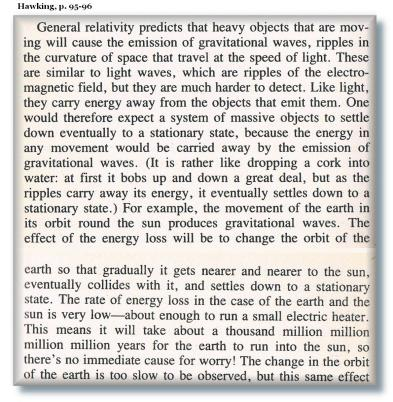Gravitational Waves & General Relativity
Version 1 - 22 May 2016 - Jonathan Ainsley Bain - www.Flight-Light-and-Spin.com
This is an extract summary - Chapter 28 in full is here:
Gravitational Waves & General Relativity.pdf

 1 2 3 4 5 6 7

pg 1

 This article is a computational analysis of the theory of gravitational waves; as expressed within Einstein’s Theory of General Relativity and the wider realm of Astrophysics. Essentially a critique, this study has been written for the purpose of explaining the unobvious challenges faced in building graphically dynamic evolutionary computer models. These models compute the theoretical functionality of gravitational waves in the celestial paradigms of solar system formation and galaxy formation. This script is intended to be interpreted by computer programmers, philosophers, physicists, mathematicians, psychologists and the curious public. It is thus expressed in ordinary language devoid of jargon as much as is possible. A simplified explanation of gravitational waves can be described thus: If a massive object four light-years away shifts position, it will be four years before its gravitational force shifts that same affect upon us. So the force of gravity is said to propagate at the velocity of light. Gravity is then also defined as the emission of moving particles called gravitons, which move away from their source object at the velocity of light. The source object obtains the energy for the emission of these gravitons from its movement through space; and thus the object must slow down as its kinetic energy decreases after emitting gravitational waves. Only after the gravitational wave reaches the destination object, is the familiar pull of gravity then applied. In ‘A Brief History of Time’, Hawking gives a more detailed explanation:Numerous logical contradictions have been encountered in constructing computer models for depicting gravitational waves. However, if you observe the following scenarios, then you will see how these apparent contradictions are resolved in such a way as to give a clearer understanding of Astrophysical structures in the Cosmos. Often I will label something a contradiction – but only temporarily – as our universe is indeed a logical place without contradictions. If you are patient then you will encounter a deeper understanding of what it takes to compute such ideas. § The most obvious problem is the event horizon of the black-hole. General Relativity claims that time itself slows down with increased gravity. Stephen Hawking and most other Astrophysicists claim that the surface gravity of the black-hole is so strong that anything trapped in this event horizon supposedly experiences infinite time. A photon or any other object traveling at any velocity within the bounds of the event horizon at the edge of the black-hole cannot escape. This is because here time has slowed to an absolute standstill. So how are gravitational waves expected to escape the event horizon? The graviton moves at the velocity of light, which being a velocity, requires time to move. But time has stopped. The black-hole would then be unable to emit anything, including gravity which is emitted at a velocity - which thus requires time to not be infinitely slow. (This question is labeled Scenario 2A in the Full Article)

pg 1

This is an extract summary
The full Chapter 28 is here:

Gravitational-Waves+General-Relativity.pdf